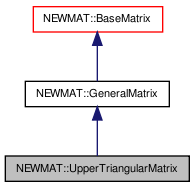# NEWMAT::UpperTriangularMatrix Class Reference

`#include <newmat.h>`

Inheritance diagram for NEWMAT::UpperTriangularMatrix:[legend]

## Detailed Description

Definition at line 708 of file newmat.h.

List of all members.

## Public Member Functions

UpperTriangularMatrix ()
~UpperTriangularMatrix ()
UpperTriangularMatrix (ArrayLengthSpecifier)
void operator= (const BaseMatrix &)
UpperTriangularMatrixoperator= (const UpperTriangularMatrix &m)
UpperTriangularMatrix (const BaseMatrix &)
UpperTriangularMatrix (const UpperTriangularMatrix &gm)
UpperTriangularMatrixoperator= (Real f)
Real & operator() (int, int)
Real & element (int, int)
Real operator() (int, int) const
Real element (int, int) const
MatrixType Type () const
GeneralMatrixMakeSolver ()
void Solver (MatrixColX &, const MatrixColX &)
LogAndSign LogDeterminant () const
Real Trace () const
void GetRow (MatrixRowCol &)
void GetCol (MatrixRowCol &)
void GetCol (MatrixColX &)
void RestoreCol (MatrixRowCol &)
void RestoreCol (MatrixColX &c)
void NextRow (MatrixRowCol &)
void ReSize (int)
void ReSize (const GeneralMatrix &A)
MatrixBandWidth BandWidth () const
void operator+= (const UpperTriangularMatrix &M)
void operator-= (const UpperTriangularMatrix &M)
void operator+= (Real f)
void operator-= (Real f)
void swap (UpperTriangularMatrix &gm)

## Private Member Functions

GeneralMatrixImage () const

## Constructor & Destructor Documentation

 NEWMAT::UpperTriangularMatrix::UpperTriangularMatrix ( )

Definition at line 712 of file newmat.h.

Referenced by Image().

 NEWMAT::UpperTriangularMatrix::~UpperTriangularMatrix ( )

Definition at line 713 of file newmat.h.

 NEWMAT::UpperTriangularMatrix::UpperTriangularMatrix ( ArrayLengthSpecifier n )

Definition at line 59 of file newmat4.cpp.

 NEWMAT::UpperTriangularMatrix::UpperTriangularMatrix ( const BaseMatrix & M )

Definition at line 129 of file newmat4.cpp.

 NEWMAT::UpperTriangularMatrix::UpperTriangularMatrix ( const UpperTriangularMatrix & gm )

Definition at line 718 of file newmat.h.

## Member Function Documentation

 MatrixBandWidth NEWMAT::UpperTriangularMatrix::BandWidth ( ) const` [virtual]`

Reimplemented from NEWMAT::BaseMatrix.

Definition at line 448 of file newmat4.cpp.

 Real NEWMAT::UpperTriangularMatrix::element ( int m, int n ) const

Definition at line 701 of file newmat6.cpp.

 Real & NEWMAT::UpperTriangularMatrix::element ( int m, int n )

Definition at line 693 of file newmat6.cpp.

 void NEWMAT::UpperTriangularMatrix::GetCol ( MatrixColX & mrc ) ` [virtual]`

Implements NEWMAT::GeneralMatrix.

Definition at line 304 of file newmat3.cpp.

 void NEWMAT::UpperTriangularMatrix::GetCol ( MatrixRowCol & mrc ) ` [virtual]`

Implements NEWMAT::GeneralMatrix.

Definition at line 281 of file newmat3.cpp.

 void NEWMAT::UpperTriangularMatrix::GetRow ( MatrixRowCol & mrc ) ` [virtual]`

Implements NEWMAT::GeneralMatrix.

Definition at line 273 of file newmat3.cpp.

 GeneralMatrix * NEWMAT::UpperTriangularMatrix::Image ( ) const` [private, virtual]`

Reimplemented from NEWMAT::GeneralMatrix.

Definition at line 774 of file newmat4.cpp.

 LogAndSign NEWMAT::UpperTriangularMatrix::LogDeterminant ( ) const` [virtual]`

Reimplemented from NEWMAT::GeneralMatrix.

Definition at line 668 of file newmat8.cpp.

 GeneralMatrix* NEWMAT::UpperTriangularMatrix::MakeSolver ( ) ` [virtual]`

Reimplemented from NEWMAT::GeneralMatrix.

Definition at line 730 of file newmat.h.

 void NEWMAT::UpperTriangularMatrix::NextRow ( MatrixRowCol & mrc ) ` [virtual]`

Reimplemented from NEWMAT::GeneralMatrix.

Definition at line 330 of file newmat3.cpp.

 Real NEWMAT::UpperTriangularMatrix::operator() ( int m, int n ) const

Definition at line 159 of file newmat6.cpp.

 Real & NEWMAT::UpperTriangularMatrix::operator() ( int m, int n )

Definition at line 47 of file newmat6.cpp.

 void NEWMAT::UpperTriangularMatrix::operator+= ( Real f )

Reimplemented from NEWMAT::GeneralMatrix.

Definition at line 745 of file newmat.h.

 void NEWMAT::UpperTriangularMatrix::operator+= ( const UpperTriangularMatrix & M )

Definition at line 743 of file newmat.h.

Referenced by operator+=().

 void NEWMAT::UpperTriangularMatrix::operator-= ( Real f )

Reimplemented from NEWMAT::GeneralMatrix.

Definition at line 746 of file newmat.h.

 void NEWMAT::UpperTriangularMatrix::operator-= ( const UpperTriangularMatrix & M )

Definition at line 744 of file newmat.h.

Referenced by operator-=().

 UpperTriangularMatrix& NEWMAT::UpperTriangularMatrix::operator= ( Real f )

Reimplemented from NEWMAT::GeneralMatrix.

Definition at line 719 of file newmat.h.

 UpperTriangularMatrix& NEWMAT::UpperTriangularMatrix::operator= ( const UpperTriangularMatrix & m )

Definition at line 716 of file newmat.h.

 void NEWMAT::UpperTriangularMatrix::operator= ( const BaseMatrix & X )

Definition at line 394 of file newmat6.cpp.

Referenced by operator=().

 void NEWMAT::UpperTriangularMatrix::ReSize ( const GeneralMatrix & A ) ` [virtual]`

Reimplemented from NEWMAT::GeneralMatrix.

Definition at line 344 of file newmat4.cpp.

 void NEWMAT::UpperTriangularMatrix::ReSize ( int nr )

Definition at line 263 of file newmat4.cpp.

Referenced by ReSize().

 void NEWMAT::UpperTriangularMatrix::RestoreCol ( MatrixColX & c ) ` [virtual]`

Reimplemented from NEWMAT::GeneralMatrix.

Definition at line 738 of file newmat.h.

Referenced by RestoreCol().

 void NEWMAT::UpperTriangularMatrix::RestoreCol ( MatrixRowCol & mrc ) ` [virtual]`

Reimplemented from NEWMAT::GeneralMatrix.

Definition at line 320 of file newmat3.cpp.

 void NEWMAT::UpperTriangularMatrix::Solver ( MatrixColX & mcout, const MatrixColX & mcin ) ` [virtual]`

Reimplemented from NEWMAT::GeneralMatrix.

Definition at line 51 of file newmat7.cpp.

 void NEWMAT::UpperTriangularMatrix::swap ( UpperTriangularMatrix & gm )

Definition at line 747 of file newmat.h.

Referenced by NEWMAT::swap().

 Real NEWMAT::UpperTriangularMatrix::Trace ( ) const` [virtual]`

Reimplemented from NEWMAT::BaseMatrix.

Definition at line 576 of file newmat8.cpp.

 MatrixType NEWMAT::UpperTriangularMatrix::Type ( ) const` [virtual]`

Implements NEWMAT::GeneralMatrix.

Definition at line 429 of file newmat4.cpp.

The documentation for this class was generated from the following files:

 newmat11b Generated Mon May 9 04:54:19 2016 by Doxygen 1.6.3